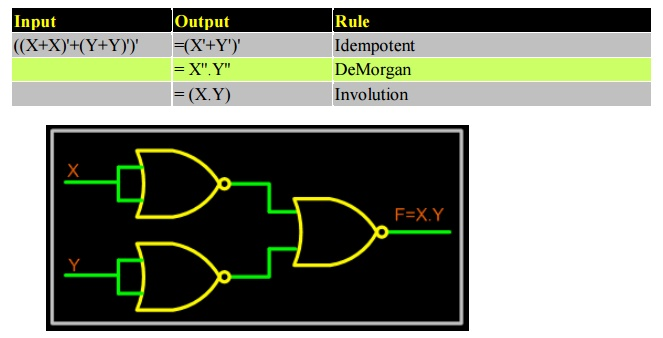Home | | Digital Electronics | NAND-NOR Implementations

# NAND-NOR Implementations

Universal gates are the ones which can be used for implementing any gate like AND, OR and NOT, or any combination of these basic gates; NAND and NOR gates are universal gates.

NANDâ€“NOR IMPLEMENTATIONS

Universal gates are the ones which can be used for implementing any gate like AND, OR and NOT, or any combination of these basic gates; NAND and NOR gates are universal gates. But there are some rules that need to be followed when implementing NAND or NOR based gates.

To facilitate the conversion to NAND and NOR logic, we have two new graphic symbols for these gates.

NAND GateNOR GateRealization of logic function using NAND gates

Any logic function can be implemented using NAND gates. To achieve this, first the logic function has to be written in Sum of Product (SOP) form. Once logic function is converted to SOP, then is very easy to implement using NAND gate. In other words any logic circuit with AND gates in first level and OR gates in second level can be converted into a NAND-NAND gate circuit.

Consider the following SOP expression

F = W.X.Y + X.Y.Z + Y.Z.W

The above expression can be implemented with three AND gates in first stage and one OR gate in second stage as shown in figure.If bubbles are introduced at AND gates output and OR gates inputs (the same for NOR gates), the above circuit becomes as shown in figure.Now replace OR gate with input bubble with the NAND gate. Now we have circuit which is fully implemented with just NAND gates.Realization of logic gates using NAND gates

Implementing an inverter using NAND gateImplementing AND using NAND gatesImplementing OR using NAND gatesImplementing NOR using NAND gatesRealization of logic function using NOR gates

Any logic function can be implemented using NOR gates. To achieve this, first the logic function has to be written in Product of Sum (POS) form. Once it is converted to POS, then it's very easy to implement using NOR gate. In other words any logic circuit with OR gates in first level and AND gates in second level can be converted into a NOR-NOR gate circuit.

Consider the following POS expression

F = (X+Y) . (Y+Z)

The above expression can be implemented with three OR gates in first stage and one AND gate in second stage as shown in figure.If bubble are introduced at the output of the OR gates and the inputs of AND gate, the above circuit becomes as shown in figure.Now replace AND gate with input bubble with the NOR gate. Now we have circuit which is fully implemented with just NOR gates.Realization of logic gates using NOR gates

Implementing an inverter using NOR gateImplementing AND using NOR gatesImplementing OR using NOR gatesImplementing NAND using NOR gatesStudy Material, Lecturing Notes, Assignment, Reference, Wiki description explanation, brief detail
Digital Electronics : Minimization Techniques and Logic Gates : NAND-NOR Implementations |